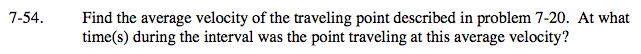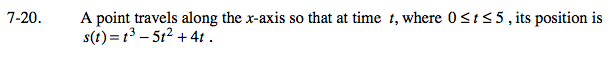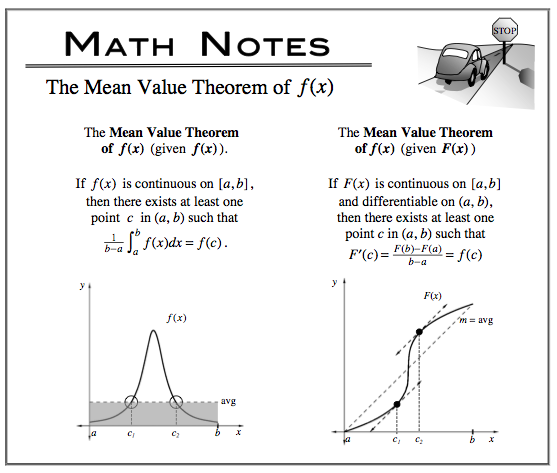### Home > CALC > Chapter 7 > Lesson 7.1.5 > Problem7-54

7-54.This is an application of the Mean Value Theorem of f(x) (given F(x)).
In other words, where on the interval [0,5] is the slope of the tangent the same as the slope of the secant?

Find where IROC = AROC

real velocity = average velocity
slope of tangent = slope of secant
Solve for t to find out WHERE the equation above holds true.